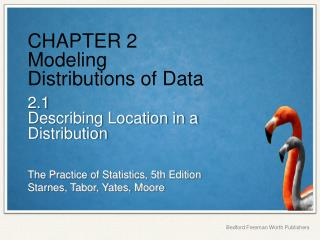DownloadDownload PresentationCHAPTER 2 Modeling Distributions of Data

# CHAPTER 2 Modeling Distributions of Data

Télécharger la présentation## CHAPTER 2 Modeling Distributions of Data

- - - - - - - - - - - - - - - - - - - - - - - - - - - E N D - - - - - - - - - - - - - - - - - - - - - - - - - - -
##### Presentation Transcript

1. CHAPTER 2Modeling Distributions of Data 2.1Describing Location in a Distribution

2. Describing Location in a Distribution • FIND and INTERPRET the percentile of an individual value within a distribution of data. • ESTIMATE percentiles and individual values using a cumulative relative frequency graph. • FIND and INTERPRET the standardized score (z-score) of an individual value within a distribution of data. • DESCRIBE the effect of adding, subtracting, multiplying by, or dividing by a constant on the shape, center, and spread of a distribution of data.

3. Measuring Position: Percentiles One way to describe the location of a value in a distribution is to tell what percent of observations are less than it. The pth percentile of a distribution is the value with p percent of the observations less than it. Example Jenny earned a score of 86 on her test. How did she perform relative to the rest of the class? 6 7 7 2334 7 5777899 8 00123334 8 569 9 03 6 7 7 2334 7 5777899 8 00123334 8 569 9 03 Her score was greater than 21 of the 25 observations. Since 21 of the 25, or 84%, of the scores are below hers, Jenny is at the 84th percentile in the class’s test score distribution.

4. Cumulative Relative Frequency Graphs A cumulative relative frequency graph displays the cumulative relative frequency of each class of a frequency distribution.

5. Measuring Position: z-Scores A z-score tells us how many standard deviations from the mean an observation falls, and in what direction. If x is an observation from a distribution that has known mean and standard deviation, the standardized score of x is: A standardized score is often called a z-score. Example Jenny earned a score of 86 on her test. The class mean is 80 and the standard deviation is 6.07. What is her standardized score?

6. Transforming Data Transforming converts the original observations from the original units of measurements to another scale. Transformations can affect the shape, center, and spread of a distribution. Effect of Adding (or Subtracting) a Constant • Adding the same number a to (subtracting a from) each observation: • adds a to (subtracts a from) measures of center and location (mean, median, quartiles, percentiles), but • Does not change the shape of the distribution or measures of spread (range, IQR, standard deviation).

7. Transforming Data Example Examine the distribution of students’ guessing errors by defining a new variable as follows: error = guess − 13 That is, we’ll subtract 13 from each observation in the data set. Try to predict what the shape, center, and spread of this new distribution will be.

8. Transforming Data Transforming converts the original observations from the original units of measurements to another scale. Transformations can affect the shape, center, and spread of a distribution. Effect of Multiplying (or Dividing) by a Constant • Multiplying (or dividing) each observation by the same number b: • multiplies (divides) measures of center and location (mean, median, quartiles, percentiles)by b • multiplies (divides) measures of spread (range, IQR, standard deviation) by |b|, but • does not change the shape of the distribution

9. Transforming Data Example Because our group of Australian students is having some difficulty with the metric system, it may not be helpful to tell them that their guesses tended to be about 2 to 3 meters too high. Let’s convert the error data to feet before we report back to them. There are roughly 3.28 feet in a meter.

10. Describing Location in a Distribution • FIND and INTERPRET the percentile of an individual value within a distribution of data. • ESTIMATE percentiles and individual values using a cumulative relative frequency graph. • FIND and INTERPRET the standardized score (z-score) of an individual value within a distribution of data. • DESCRIBE the effect of adding, subtracting, multiplying by, or dividing by a constant on the shape, center, and spread of a distribution of data.